# Appendix: Accelerating convergence for other series

We can easily play the same game with the other series mentioned in this article. For example take the Gregory/Leibnitz series. There is a subtlety with this case as this is an alternating series which, as you add it up, takes values which oscillate above and below the value of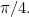To avoid this we will define the sum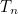by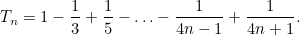This sum is always slightly greater than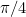. Then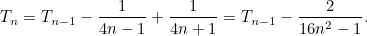As before we will assume that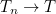and that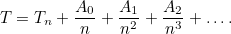Comparing the two expressions forand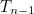we get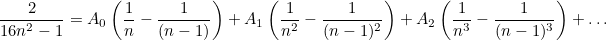Again, as before, we can find the values of the terms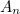by expanding out each of these fractions and comparing powers of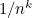when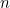is large. This is slightly more complicated than last time an we must use the result that for large values of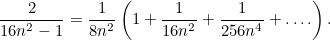Doing this we find that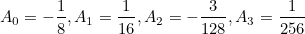so that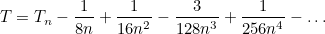As before we will compare the approximations to the sum given byand by the corrections to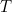given by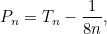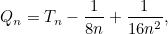and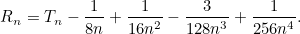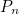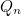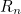1 0.8666666666666 0.741666666666667 0.804166666666667 0.784635416666667 5 0.8080789523513 0.783078952351398 0.785578952351398 0.785397702351398 10 0.7972961955693 0.784796195569399 0.785421195569399 0.785398148694399

Again if we compare these values to the true value of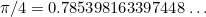then we see excellent agreement, with real economy of effort. In fact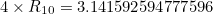which is a far better estimate for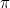than the one that we obtained earlier by adding up 100 terms of the same series.

Back to main article